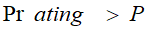# ASME PTC 2010 - Intergraph Smart Instrumentation - 13.1 - Reference - Hexagon PPM

## Integraph Smart Instrumentation Sizing Equations

Language
English (United States)
Product
Intergraph Smart Instrumentation
Search by Category
Reference
Smart Instrumentation Version
13.1
1. Calculate mounting factor:

*L [m]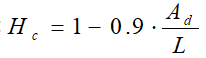• Other connection types: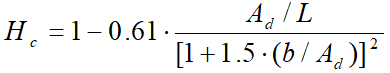2. Calculate Reynolds number: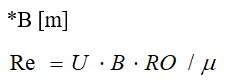3. Calculate Strouhal number: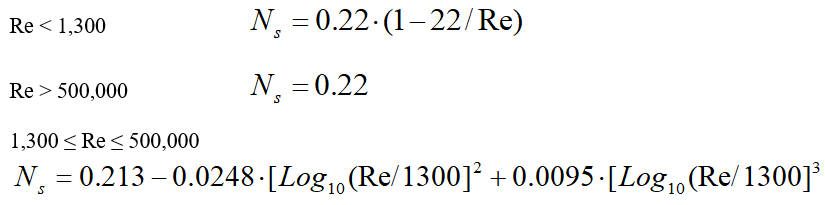4. Calculate natural frequency:

*L [m], B[m,] E[Pag]

1. Calculate average outside diameter:

• Tapered thermowell: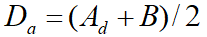• Other thermowells: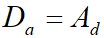1. Calculate approximate natural frequency: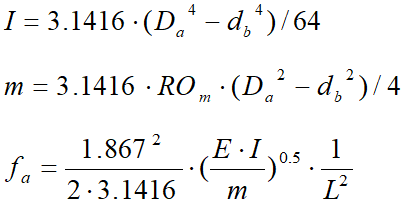2. Calculate correction factor Hf

• Tapered and straight thermowell: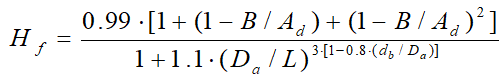• Stepped thermowell: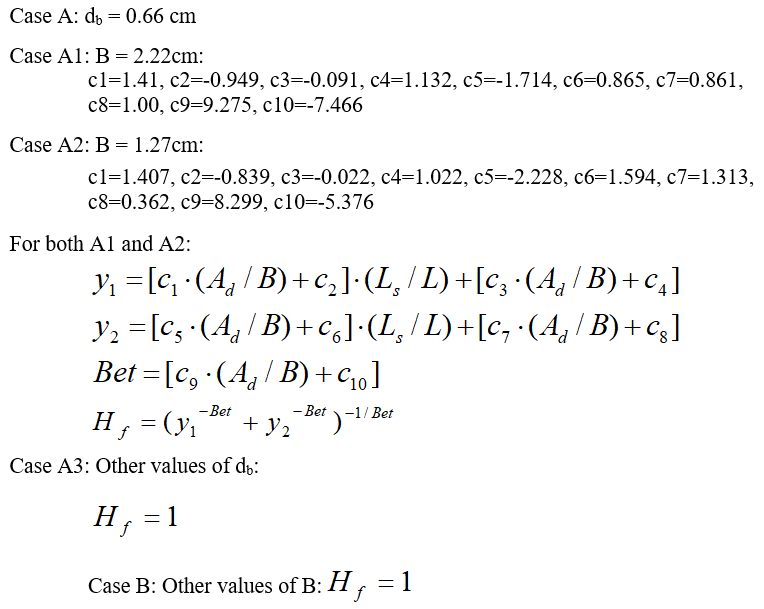1. Calculate correction factor Haf: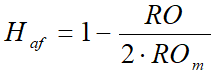2. Calculate correction factor Has: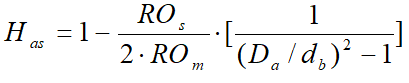3. Calculate natural frequency with ideal support: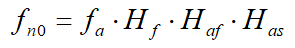4. Calculate natural frequency of the mounted thermowell: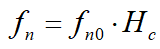5. Calculate Vortex shedding frequency

*B[m]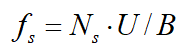6. Calculate Scruton number:

*B[m]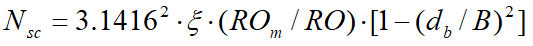7. Calculate miscellaneous parameters:

*B[m], L[m], E[Pag

1. Parameter Gsp

• Tapered and straight thermowell: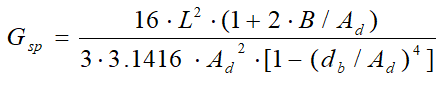• Stepped thermowell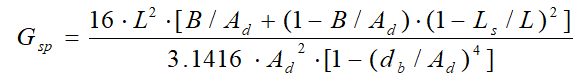1. Parameter Grd (for stepped thermowell only):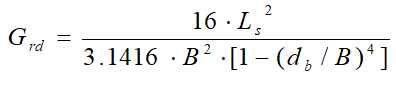2. Stress concentration factor: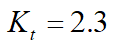• Other connections: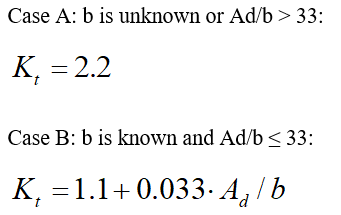1. Stress concentration factor at support plane (for stepped thermowell only):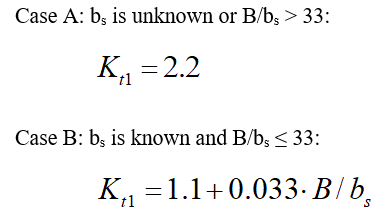2. Temperature de-rating factor: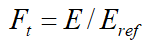8. Calculate Frequency Limit

• Low density gases: Nsc >2.5 and Tr ,100000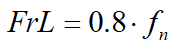• General case: Other values of Nsc and Re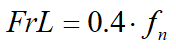9. Compliance with the frequency limit requirement:

1. Initial evaluation of compliance:

• The thermowell is in compliance with the frequency limit requirement if;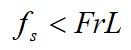Go to step 10.

• The thermowell is non-compliant with the frequency limit requirement if;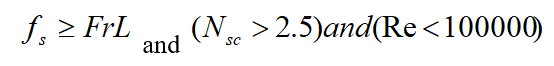Go to step 10.

1. Evaluate compliance with cyclic stress limit for general case:

• Calculate velocity for in-line resonance:

1.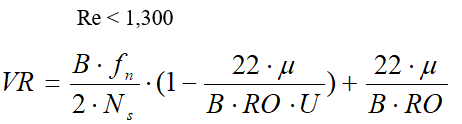2.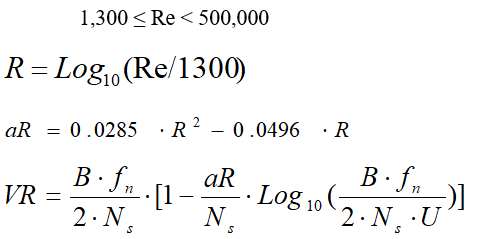3.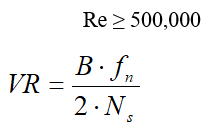1. Calculate cyclic drag stress at the root: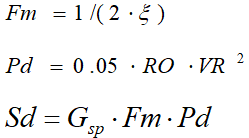2. Calculate combined drag and lift stresses: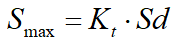3. Calculate fatigue stress limit: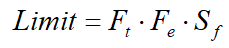4. Compliance with cyclic stress limit:

• Tapered and straight thermowell:

• Not compliant with the cyclic stress limit and frequency limit if;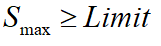Go to step 10.

• Complies with the cyclic stress limit if;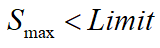Set new frequency limit: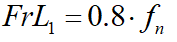Go to step 9g

• Stepped thermowell:

• Not compliant with the cyclic stress limit and frequency limit if;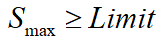Go to step 10.

• Case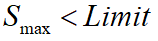Calculate: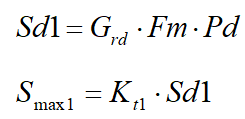• Not compliant with the cyclic stress limit and frequency limit if;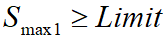Go to step 10.

• Complies with the cyclic stress limit if;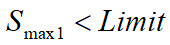Set new frequency limit: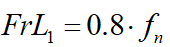Go to step 9-g.

1. Re-evaluate compliance with the frequency limit requirement if cyclic stress is acceptable:

• The thermowell is in compliance with the frequency limit requirement if;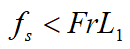• The thermowell is in non-compliant with the frequency limit requirement if;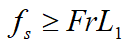10. Compliance with the static stress limit requirement:

*B[m], P[Pag], S[Pag]

1. Calculate radial, tangential, and axial stress due to external pressure: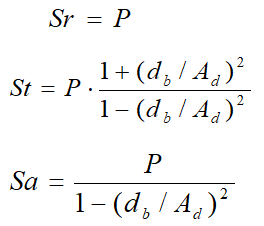2. Calculate steady-state drag stress at the root: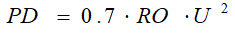3. Calculate steady-state stress due to the drag force: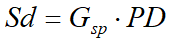4. Calculate maximum stress: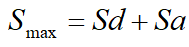5. Calculate left-hand side of the Von Mises criteria: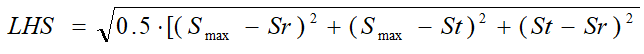6. Calculate right-hand side of the Von Mises criteria: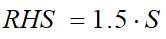Compliance

• Tapered and straight thermowell:

• Not compiled with the static stress limit if;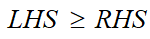• Complies with the static limit if;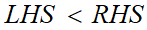• Stepped thermowell:

• Not compiled with the static stress limit if;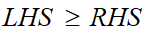• Case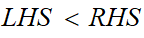Calculate: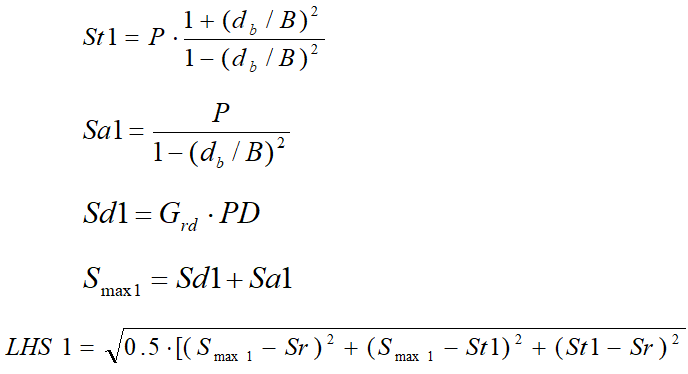• Not compliant with the static stress limit if;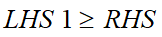• Complies with the static stress limit if;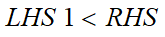11. Compliance with the Dynamic Stress Limit requirement

Calculate parameters: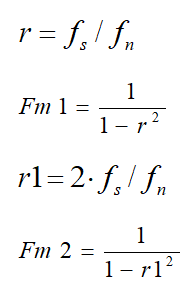12. Calculate drag and lift stresses at the root: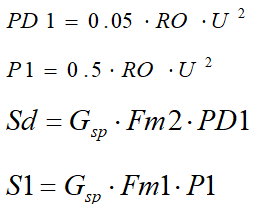13. Calculate combined drag and lift stresses: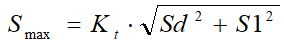14. Calculate fatigue stress limit: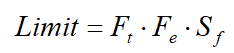Compliance

• Tapered and straight thermowell

• Not compliant with the dynamic stress limit if;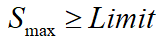• Complies with the dynamic stress limit if;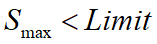• Stepped thermowell

• Not compliant with the dynamic stress limit if;Go to Compliance with the Hydrostatic Pressure Limit requirement section in this topic

•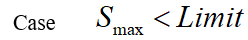• Calculate: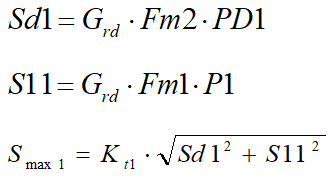• Not compliant with the dynamic stress limit if;• Complies with the dynamic stress limit if;15. Compliance with the Hydrostatic Pressure Limit requirement

*B[m], P[Pag], S[Pag]

16. Calculate pressure rating for the shank: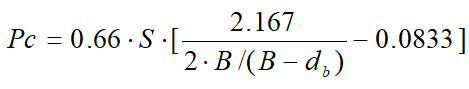17. Calculate external pressure rating for the tip: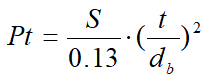18. Calculate pressure rating of the thermowell: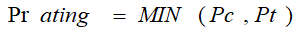19. Compliance

• Not compliant with the hydrostatic pressure limit if;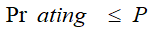• Compliant with hydrostatic pressure limit if;